## 2.14 PEDESTRIAN CROSSING PROBLEM

In this section we study a problem involving the timing of a pedestrian street-crossing light. The problem provides a good illustration of many of the probabilistic modeling techniques that we have reviewed in this chapter, particularly the Poisson process. It also provides an example of applying mathematical modeling to the evaluation of technological alternatives in an urban setting.

The problem is as follows. Pedestrians approach from the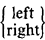side of the crossing in a Poisson manner with average arrival rate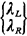arrivals per minute (Figure 2.14). Each pedestrian then waits until a light is flashed, at which time all waiting pedestrians must cross. We refer to each time the light is flashed as a "dump" and assume that a dump takes zero time (i.e., pedestrians cross instantly). Assume that the left and right arrival processes are independent.

We wish to analyze three possible decision rules for operating the light:

 Rule A: Dump every T minutes. Rule B: Dump whenever the total number of waiting pedestrians equals N0. Rule C: Dump whenever the first pedestrian to arrive after the previous dump has waited T0 minutes.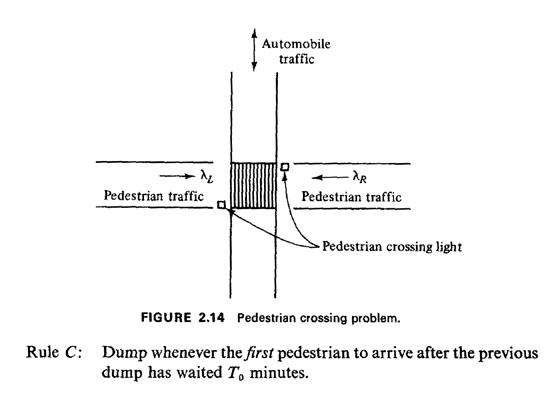Presumably, implementation of each rule requires a particular type of technology with its attendant costs, and thus it is important to determine the operating characteristics of each in order to understand tradeoffs between performance and cost.

For each decision rule, determine:

 1 The expected number of pedestrians crossing left to right on any dump. 2 The probability that zero pedestrians cross left to right on any particular dump. 3 The pdf for the time between dumps. 4 The expected time that a randomly arriving pedestrian must wait until crossing. 5 The expected time that a randomly arriving observer, who is not a pedestrian, will wait until the next dump.
The last two parts to this question will provide a good motivation for our more general study of queues in Chapter 4.

We answer each part in sequence, analyzing each of the three decision rules as we proceed.

1. Let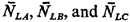be the expected number of pedestrians crossing left to right on any dump for each of the three decision rules A, B, and C, respectively.

For interdump times fixed at T minutes, we simply require the expected number of Poisson arrivals in an interval [0, T], which gives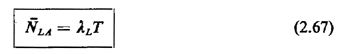If the decision rule is to dump whenever there are exactly N0 waiting pedestrians, obviously the total number of pedestrians crossing will be equal to N0 However, the probability that any particular one is a left-to-right crossing pedestrian is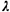L/(L +R), and the type of each successive pedestrian is chosen independently. Thus, we may think of each dump as N0 independent Bernoulli trials each having "success" probabilityL/(L +R). Clearly, the expected number of left-to-right crossing pedestrians in this case is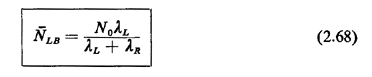For rule C, the expected number of left-to-right crossing pedestrians during the pedestrian-initiated waiting period of duration T0 minutes isLT0. However, we must also count the first arriving pedestrian who initiates this waiting period; he has a probability equal toL/(L +R of being a "left-to-right" pedestrian. Thus, using a "left-pedestrian" indicator random variable, added to a Poisson random variable, we obtain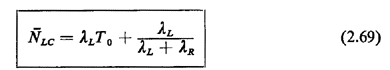2. Let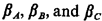be the three desired probabilities for decision rules A, B, and C, respectively.

For decision rule A,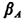is simply the probability of zero Poisson arrivals in a fixed interval of length T,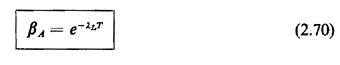For decision rule B, the probability that zero of the N0 crossing pedestrians are left-to-right pedestrians is equal to the probability that N0 successive independent Bernoulli trials yield a right-to-left pedestrian. Thus,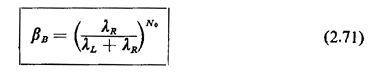For decision rule C,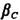is equal to the probability that the first arriving pedestrian is a right-to-left pedestrian and that no left-to-right pedestrians arrive in the following T0 minutes. By independence, the probability of this intersection of events is equal to the product of the individual probabilities,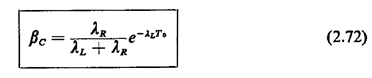3. Let the three desired pdf's be fXA(.), fXB(.), and fXC(.), respectively. Since we are finding the pdf's for the time between dumps and since the decision rules utilize information on the total number of arriving pedestrians (and rule A utilizes no information on arriving pedestrians), we need only consider in this part the pooled Poisson process having arrival rateL +R.

The first pdf is simply the unit impulse located at T; thus,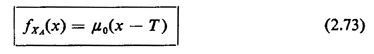The second pdf corresponds to the N0th-order interarrival time pdf for a Poisson process with (pooled) arrival rateL +R. By (2.57), this is simply the N0th-order Erlang pdf,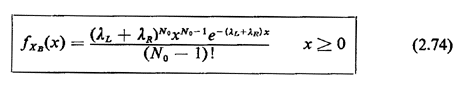For the third pdf we use the fact that the time between dumps Xc can be expressed as

XC = time until first pedestrian arrives + T0

But the time until the first pedestrian arrives is equal to the first-order interarrival time in a (pooled) process with rateL +R, and this is simply a negative exponential pdf with mean (L +R)-1. Thus, the pdf for XC is simply the negative exponential pdf shifted to the right by T0 minutes,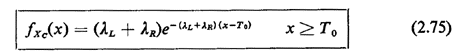4. Let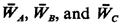be the three desired expected waiting times of a randomly arriving pedestrian. The reasoning here, while the most advanced in the problem, will provide some beginning insights into the theory ofqueues, which we study in earnest in Chapters 4 and 5. As in part 3, here we need only study the pooled process having rateL +R.

For rule A, in which a dump occurs every T minutes, we can correctly reason that his or her arrival time in the interval [0, T] is uniformly distributed over the interval, and thus the waiting time until the end of the interval is uniformly distributed, implying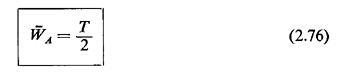To analyze rule B we must be a bit more careful. A randomly arriving pedestrian is equally likely to be the first to arrive since the last dump, the second to arrive, . . ., the N0th to arrive. Thus, the probability that a randomly arriving pedestrian is the kth to arrive since the last dump is equal to 1/N0 for k = 1, 2, . . . , N0. Given that he or she is the kth to arrive, his or her conditional waiting time until the next dump is distributed as an (N0 - k)order Erlang random variable with parameterL +R (where we define a zero-order Erlang random variable to always assume the experimental value zero). Thus, the conditional mean waiting time of the kth arriving pedestrian is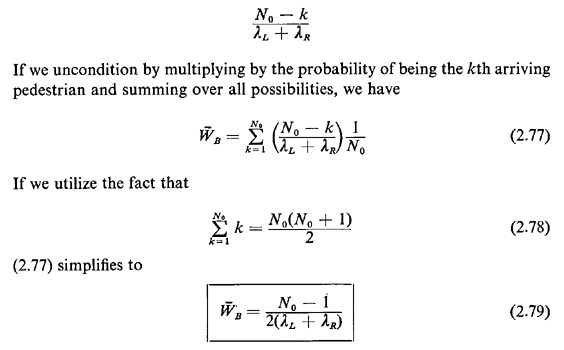Does this make sense intuitively?

To analyze rule C, let us condition on the total number of pedestrians who arrive during the interval of length T0 initiated by the first arriving pedestrian. Clearly, the first arriving pedestrian has an expected wait of T0 minutes and any other arriving pedestrian prior to the dump has an expected wait of T0/2 minutes. We seek to combine these two conditional expected waits in an appropriate manner. Call (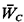|k) the conditional expected wait of a randomly arriving pedestrian, given that exactly k pedestrians arrive in the associated interval of length T0. Then clearly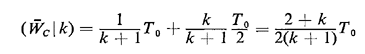To find now the expected unconditional waiting time,, per pedestrian, we must multiply by the probability that a randomly chosen pedestrian has crossed the street in a group of k + 1 pedestrians. This is a case of random incidence and the probability in question [use the discrete analogue of (2.63)] is given by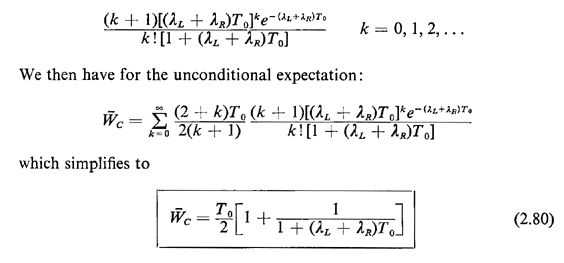We can check the reasonableness of this result for two limiting cases: (1) as T0 becomes large compared to the mean passenger interarrival time (L +R)-1, the mean wait approaches one half the mean interdump time, a result in agreement with (2.66) for the case in which the variance of the interdump time is small compared to the square of the mean; and (2) as T0 becomes very small,approaches T, (which is also expected, since in that case nearly all crossing pedestrians are first arriving pedestrians).

We could obtain (2.80) by another argument, based on "perturbation random variables," as formalized in Section 3.8. Roughly, the argument goes as follows. Each pedestrian must incur an average wait of T0/2 minutes. In addition, there is a certain probability that a randomly arriving pedestrian must incur an additional mean wait of T0/2 minutes; this probability is equal to the probability of being the first arriving pedestrian. Thus, we could writeas the sum of T0/2 plus a perturbation term, as follows: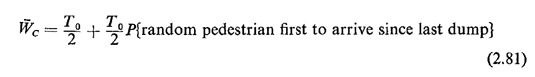To compute the probability in the perturbation term, consider a very long period of time during which N dumps, where N is a large number, have taken place. This means that N "first pedestrians" have arrived and an expected number of N(L +R)T0 pedestrians arrived during the T0-long intervals that follow the arrival of the first pedestrian. Thus,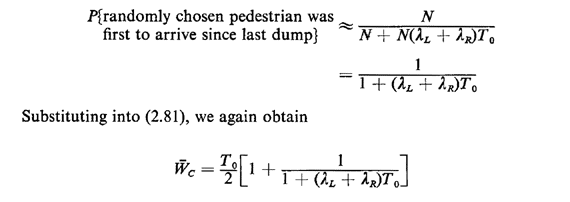Note that the result above has been argued quite informally, in keeping with the informality of the perturbation argument.

5. This part is considerably easier than part 4. However, it is instructive to compare the answers to those in part 4 for each of the three decision rules. In part 4, the randomly arriving person is a pedestrian and he or she thus "disturbs" the system. In this part the randomly arriving person is strictly an observer, and thus he or she does not affect the system. Call the times desired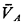,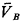,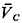for the three respective decision rules.

All we need do to obtain the three required answers is apply (2.66) derived for random incidence. Recalling that equation, in the context of this problem, it states that the expected time until the next dump (from the moment of the observer's arrival) is equal to one half the sum of the mean squared and the variance of the interdump times, divided by the mean. For instance, for decision rule A we have E[XA] = T and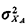= 0, implying that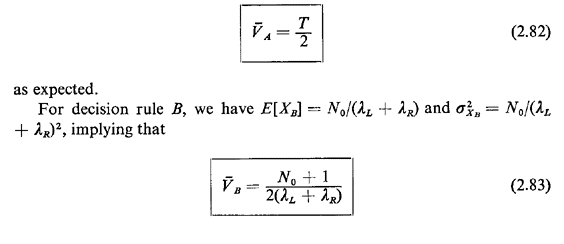Note that this result is similar to that derived in part 4, except that the "+ 1" here is replaced by a "-1." Does this make sense intuitively? Can you derive (2.83) along the lines argued in part 4 to confirm this result? For decision rule C, we have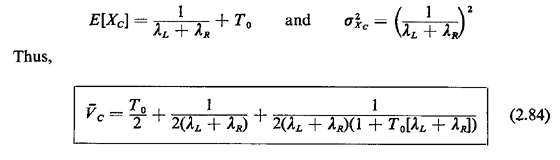This result, too, checks with our intuition in two limiting cases: as T00,1/(L +R), implying that the expected time until the next dump is equal to the expected time of arrival of the next pedestrian. As T0 becomes large,[T0 + (L +R)-1]/2 which is one half the expected interdump time; this result checks with (2.66) for the case in which the variance of the interdump time is negligibly small in comparison to the square of the mean.

This completes our analysis of the pedestrian crossing problem. In addition to providing us with a good example of probabilistic modeling as applied to an urban service system, it has pointed to directions we wish to pursue in Chapters 3-5.

Example 8: Pedestrian Crossing, One More Time

One way to evaluate the alternative decision rules is to design each system to yield the same mean pedestrian waiting time and then to determine which system has the smallest average frequency of pedestrian light flashes (a small frequency would reduce the disruption of vehicular traffic). Or, we could hold constant the frequency of pedestrian light flashes and determine which system has the smallest mean pedestrian waiting time. Intuitively, how would you rank-order the systems according to this evaluation yardstick? Can you prove your intuition mathematically? Try several typical values ofL,R and desired mean wait to observe the range and sensitivity of the results. Hint: Write an expression for the mean time between pedestrian light flashes for each of the three systems. You should then be able to express each of the pedestrian mean waiting times derived in part (4) in the form 1/2.(mean time between pedestrian light flashes) - (perturbation term). By examining the perturbation terms, you should be able to show that system A is the least preferred, system B the most preferred, and system C falls between the two. However, in comparing systems B and C, you should also consider the technological feasibility and associated cost of each of the systems.